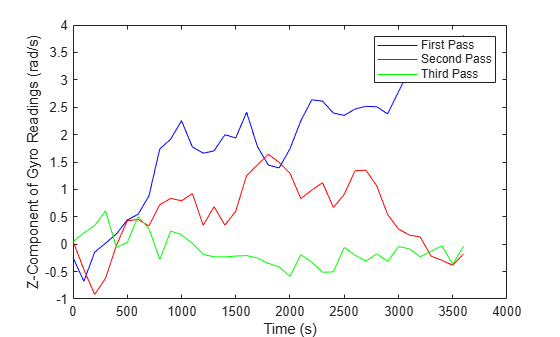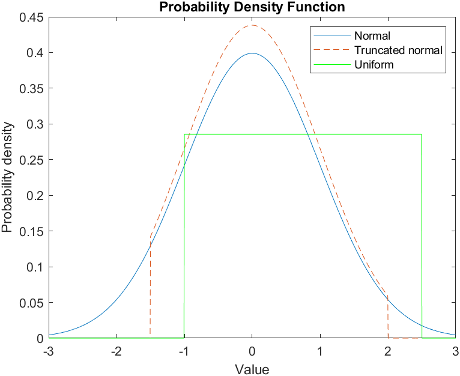perturbations

Perturbation defined on object

Description

example

perturbs = perturbations(obj) returns the list of property perturbations, perturbs, defined on the object, obj. The returned perturbs lists all the perturbable properties. If any property is not perturbed, then its corresponding Type is returned as "Null" and its corresponding Value is returned as {Null,Null}.

perturbs = perturbations(obj,property) returns the current perturbation applied to the specified property.

perturbs = perturbations(obj,property,'None') defines a property that must not be perturbed.

example

perturbs = perturbations(obj,property,'Selection',values,probabilities) defines the property perturbation offset drawn from a set of values that have corresponding probabilities.

perturbs = perturbations(obj,property,'Normal',mean,deviation) defines the property perturbation offset drawn from a normal distribution with specified mean and standard deviation.

perturbs = perturbations(obj,property,'TruncatedNormal',mean,deviation,lowerLimit,upperLimit) defines the property perturbation offset drawn from a normal distribution with specified mean, standard deviation, lower limit, and upper limit.

example

perturbs = perturbations(obj,property,'Uniform',minVal,maxVal) defines the property perturbation offset drawn from a uniform distribution on an interval [minVal, maxValue].

perturbs = perturbations(obj,property,'Custom',perturbFcn) enables you to define a custom function, perturbFcn, that draws the perturbation offset value.

Examples

collapse all

Create a waypointTrajectory object.

traj = waypointTrajectory;

Show the default perturbation properties using the perturbations method.

perturbs = perturbations(traj)
perturbs=2×3 table
Property         Type           Value
_______________    ______    __________________

"Waypoints"        "None"    {[NaN]}    {[NaN]}
"TimeOfArrival"    "None"    {[NaN]}    {[NaN]}

Create an insSensor object.

sensor = insSensor
sensor =
insSensor with properties:

MountingLocation: [0 0 0]            m
RollAccuracy: 0.2                deg
PitchAccuracy: 0.2                deg
YawAccuracy: 1                  deg
PositionAccuracy: [1 1 1]            m
VelocityAccuracy: 0.05               m/s
AccelerationAccuracy: 0                  m/s²
AngularVelocityAccuracy: 0                  deg/s
TimeInput: 0
RandomStream: 'Global stream'

Define the perturbation on the RollAccuracy property as three values with an equal possibility each.

values = {0.1 0.2 0.3}
values=1×3 cell array
{[0.1000]}    {[0.2000]}    {[0.3000]}

probabilities = [1/3 1/3 1/3]
probabilities = 1×3

0.3333    0.3333    0.3333

perturbations(sensor,'RollAccuracy','Selection',values,probabilities)
ans=7×3 table
Property                Type                        Value
_________________________    ___________    ______________________________________

"RollAccuracy"               "Selection"    {1x3 cell}    {[0.3333 0.3333 0.3333]}
"PitchAccuracy"              "None"         {[   NaN]}    {[                 NaN]}
"YawAccuracy"                "None"         {[   NaN]}    {[                 NaN]}
"PositionAccuracy"           "None"         {[   NaN]}    {[                 NaN]}
"VelocityAccuracy"           "None"         {[   NaN]}    {[                 NaN]}
"AccelerationAccuracy"       "None"         {[   NaN]}    {[                 NaN]}
"AngularVelocityAccuracy"    "None"         {[   NaN]}    {[                 NaN]}

Perturb the sensor object using the perturb function.

rng(2020)
perturb(sensor);
sensor
sensor =
insSensor with properties:

MountingLocation: [0 0 0]            m
RollAccuracy: 0.5                deg
PitchAccuracy: 0.2                deg
YawAccuracy: 1                  deg
PositionAccuracy: [1 1 1]            m
VelocityAccuracy: 0.05               m/s
AccelerationAccuracy: 0                  m/s²
AngularVelocityAccuracy: 0                  deg/s
TimeInput: 0
RandomStream: 'Global stream'

The RollAccuracy is perturbed to 0.5 deg.

Define a waypoint trajectory. By default, this trajectory contains two waypoints.

traj = waypointTrajectory
traj =
waypointTrajectory with properties:

SampleRate: 100
SamplesPerFrame: 1
Waypoints: [2x3 double]
TimeOfArrival: [2x1 double]
Velocities: [2x3 double]
Course: [2x1 double]
GroundSpeed: [2x1 double]
ClimbRate: [2x1 double]
Orientation: [2x1 quaternion]
AutoPitch: 0
AutoBank: 0
ReferenceFrame: 'NED'

Define perturbations on the Waypoints property and the TimeOfArrival property.

rng(2020);
perturbs1 = perturbations(traj,'Waypoints','Normal',1,1)
perturbs1=2×3 table
Property          Type            Value
_______________    ________    __________________

"Waypoints"        "Normal"    {[  1]}    {[  1]}
"TimeOfArrival"    "None"      {[NaN]}    {[NaN]}

perturbs2 = perturbations(traj,'TimeOfArrival','Selection',{[0;1],[0;2]})
perturbs2=2×3 table
Property           Type                     Value
_______________    ___________    _______________________________

"Waypoints"        "Normal"       {[     1]}    {[            1]}
"TimeOfArrival"    "Selection"    {1x2 cell}    {[0.5000 0.5000]}

Perturb the trajectory.

offsets = perturb(traj)
offsets=2×1 struct array with fields:
Property
Offset
PerturbedValue

The Waypoints property and the TimeOfArrival property have changed.

traj.Waypoints
ans = 2×3

1.8674    1.0203    0.7032
2.3154   -0.3207    0.0999

traj.TimeOfArrival
ans = 2×1

0
2

Create an imuSensor object and show its perturbable properties.

imu = imuSensor;
perturbations(imu)
ans=17×3 table
Property                    Type           Value
______________________________________    ______    __________________

"Accelerometer.MeasurementRange"          "None"    {[NaN]}    {[NaN]}
"Accelerometer.Resolution"                "None"    {[NaN]}    {[NaN]}
"Accelerometer.ConstantBias"              "None"    {[NaN]}    {[NaN]}
"Accelerometer.NoiseDensity"              "None"    {[NaN]}    {[NaN]}
"Accelerometer.BiasInstability"           "None"    {[NaN]}    {[NaN]}
"Accelerometer.RandomWalk"                "None"    {[NaN]}    {[NaN]}
"Accelerometer.TemperatureBias"           "None"    {[NaN]}    {[NaN]}
"Accelerometer.TemperatureScaleFactor"    "None"    {[NaN]}    {[NaN]}
"Gyroscope.MeasurementRange"              "None"    {[NaN]}    {[NaN]}
"Gyroscope.Resolution"                    "None"    {[NaN]}    {[NaN]}
"Gyroscope.ConstantBias"                  "None"    {[NaN]}    {[NaN]}
"Gyroscope.NoiseDensity"                  "None"    {[NaN]}    {[NaN]}
"Gyroscope.BiasInstability"               "None"    {[NaN]}    {[NaN]}
"Gyroscope.RandomWalk"                    "None"    {[NaN]}    {[NaN]}
"Gyroscope.TemperatureBias"               "None"    {[NaN]}    {[NaN]}
"Gyroscope.TemperatureScaleFactor"        "None"    {[NaN]}    {[NaN]}
⋮

Specify the perturbation for the NoiseDensity property of the accelerometer as a uniform distribution.

perturbations(imu,'Accelerometer.NoiseDensity', ...
'Uniform',1e-5,1e-3);

Specify the perturbation for the RandomWalk property of the gyroscope as a truncated normal distribution.

perts = perturbations(imu,'Gyroscope.RandomWalk', ...
'TruncatedNormal',2,1e-5,0,Inf);

numSamples = size(orientations);

Simulate the imuSensor three times with different perturbation realizations.

rng(2021); % For repeatable results
numRuns = 3;
colors = ['b' 'r' 'g'];
for idx = 1:numRuns

% Clone IMU to maintain original values
imuCopy = clone(imu);

% Perturb noise values
offsets = perturb(imuCopy);

% Obtain the measurements

% Plot the results
hold on;
end
xlabel('Time (s)')
legend("First Pass","Second Pass","Third Pass");
hold offInput Arguments

collapse all

Object to be perturbed, specified as an object. The objects that you can perturb include:

Perturbable property, specified as a property name. Use perturbations to obtain a full list of perturbable properties for the specified obj.

For the imuSensor System object™, you can perturb properties of its accelerometer, gyroscope, and magnetometer components. For more details, see the Perturb imuSensor Parameters example.

Perturbation offset values, specified as an n-element cell array of property values. The function randomly draws the perturbation value for the property from the cell array based on the values' corresponding probabilities specified in the probabilities input.

Drawing probabilities for each perturbation value, specified as an n-element array of nonnegative scalars, where n is the number of perturbation values provided in the values input. The sum of all elements must be equal to one.

For example, you can specify a series of perturbation value-probability pair as {x1,x2,…,xn} and {p1,p2,…,pn}, where the probability of drawing xi is pi (i = 1, 2, …,n).

Mean of normal or truncated normal distribution, specified as a scalar, vector, or matrix. The dimension of mean must be compatible with the corresponding property that you perturb.

Standard deviation of normal or truncated normal distribution, specified as a nonnegative scalar, vector of nonnegative scalars, or matrix of nonnegative scalars. The dimension of deviation must be compatible with the corresponding property that you perturb.

Lower limit of the truncated normal distribution, specified as a scalar, vector, or matrix. The dimension of lowerLimit must be compatible with the corresponding property that you perturb.

Upper limit of the truncated normal distribution, specified as a scalar, vector, or matrix. The dimension of upperLimit must be compatible with the corresponding property that you perturb.

Minimum value of the uniform distribution interval, specified as a scalar, vector, or matrix. The dimension of minVal must be compatible with the corresponding property that you perturb.

Maximum value of the uniform distribution interval, specified as a scalar, vector, or matrix. The dimension of maxVal must be compatible with the corresponding property that you perturb.

Perturbation function, specified as a function handle. The function must have this syntax:

offset = myfun(propVal)
where propVal is the value of the property and offset is the perturbation offset for the property.

Output Arguments

collapse all

Perturbations defined on the object, returned as a table of perturbation properties. The table has three columns:

• Property — Property names.

• Type — Type of perturbations, returned as "None", "Selection", "Normal", "TruncatedNormal", "Uniform", or "Custom".

• Value — Perturbation values, returned as a cell array.

collapse all

Specify Perturbation Distributions

You can specify the distribution for the perturbation applied to a specific property.

• Selection distribution — The function defines the perturbation offset as one of the specified values with the associated probability. For example, if you specify the values as [1 2] and specify the probabilities as [0.7 0.3], then the perturb function adds an offset value of 1 to the property with a probability of 0.7 and add an offset value of 2 to the property with a probability of 0.3. Use selection distribution when you only want to perturb the property with a number of discrete values.

• Normal distribution — The function defines the perturbation offset as a value drawn from a normal distribution with the specified mean and standard deviation (or covariance). Normal distribution is the most commonly used distribution since it mimics the natural perturbation of parameters in most cases.

• Truncated normal distribution — The function defines the perturbation offset as a value drawn from a truncated normal distribution with the specified mean, standard deviation (or covariance), lower limit, and upper limit. Different from the normal distribution, the values drawn from a truncated normal distribution are truncated by the lower and upper limit. Use truncated normal distribution when you want to apply a normal distribution, but the valid values of the property are confined in an interval.

• Uniform distribution — The function defines the perturbation offset as a value drawn from a uniform distribution with the specified minimum and maximum values. All the values in the interval (specified by the minimum and maximum values) have the same probability of realization.

• Custom distribution — Customize your own perturbation function. The function must have this syntax:

offset = myfun(propVal)
where propVal is the value of the property and offset is the perturbation offset for the property.

This figure shows probability density functions for a normal distribution, a truncated normal distribution, and a uniform distribution, respectively.